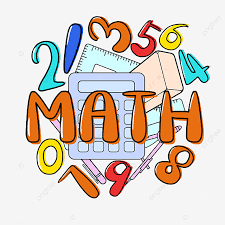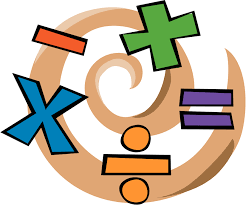# MATH

• ## 4th Grade MathVOCABULARY

 mathematical expression: A mathematical phrase with operators, letters, and/or numbers. Example:  12 + 9    5 x n    43 - x Evaluate: To find the value of an algebraic expression by replacing each letter with a given number and then simplifying. Addend: A number that is added to another number or numbers. Commutative (Ordering) Property: Changing the order does not change the sum.  Example:  5 + 6 = 6 + 5 Associative (Grouping)Property: Changing the grouping of the addends does not change the sum. Example: (4 + 5) + 2 = 4 + (5 + 2) Identity Property: The sum of 0 and a number is the same as that number. Example:  7 + 0 Estimate: An approximate answer; to find an answer that is close to the exact answer. Sum: The result of adding. Regroup: To rename ten in a place for one in the next greater place, or one in a place for ten in the next lesser place, in order to add or subtract. organized list: A list of all possible ways to do something, ordered so that you know all possiblities have been listed.

Chapter 2 Topics
 2-1:  Mathematical Expressions 2-2:  Addition Properties 2-3:  Estimate Sums 2-4:  Add Thousands 2-5:  Add Millions 2-6:  Three or More Addends 2-7:  Problem Solving: Make an Organized List

WHAT TO EXPECT...
Students will learn more advanced concepts of addition, subtraction, multiplication and division of larger digit numbers.

Students can expect to be ASSESSED in the following ways:

Classwork/Homework
Quizzes
Tests
Projects

MATH FACT FLUENCY

• I can't stress this enough, MASTERY is expected with all basic facts!
• Please make sure your child is studying their math facts. Knowing their math facts will strengthen their skills and help them understand all the new concepts they will be learning.
• Plan to practice a minimum of 3 times a week for 10 minutes a session (on-line sites and/or games).

SplashLearn
This school year I plan to use an educational program called SplashLearn to supplement their math learning.  We will utilize this program in school, but I may give additional assignments that students can work on at home.  The version we will be using will give us access to assigned practice, math facts, and progress reports.